Hostname: page-component-546b4f848f-sw5dq Total loading time: 0 Render date: 2023-06-01T16:08:08.781Z Has data issue: false Feature Flags: { "useRatesEcommerce": true } hasContentIssue false

# The onset of strongly localized thermal convection in rotating spherical shells

Published online by Cambridge University Press:  16 November 2011

*

## Abstract

A Boussinesq fluid of kinematic velocityand thermal diffusivity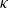is confined within a rapidly rotating shell with inner and outer sphere boundary radii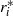and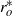, respectively. The boundaries of the shell corotate at angular velocity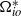and a continuously varying stratification profile is applied which is unstable in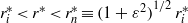and stable in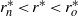. When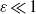, the unstable zone attached to the inner boundary is thin. As in previous small Ekman number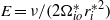studies, convection at the onset of instability takes on the familiar ‘cartridge belt’ structure, which is localized within a narrow layer adjacent to, but outside, the cylinder tangent to the inner sphere at its equator (Dormy et al. J. Fluid Mech., 2004, vol. 501, pp. 43–70), with estimated radial width of order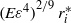. The azimuthally propagating convective columns, described by the cartridge belt, reside entirely within the unstable layer when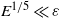, and extend from the equatorial plane an axial distance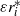along the tangent cylinder as far as its intersection with the neutrally stable spherical surface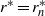. We investigate the eigensolutions of the ordinary differential equation governing the axial structure of the cartridge belt both numerically for moderate-to-small values of the stratification parameterand analytically when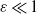. At the lowest order of the expansion in powers of, the eigenmodes resemble those for classical plane layer convection, being either steady (exchange of stabilities) or, for small Prandtl number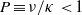, oscillatory (overstability) with a frequency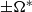. At the next order, the axial variation of the basic state removes any plane layer degeneracies. First, the exchange of stabilities modes oscillate at a low frequency causing the short axial columns to propagate as a wave with a small angular velocity, termed slow modes. Second, the magnitudes of both the Rayleigh number and frequency of the two overstable modes, termed fast modes, split. When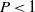the slow modes that exist at large azimuthal wavenumbers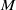make a continuous transition to the preferred fast modes at small. At all values of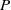the critical Rayleigh number corresponds to a mode exhibiting prograde propagation, whether it be a fast or slow mode. This feature is shared by the uniform classical convective shell models, as well as Busse’s celebrated annulus model. None of them possess any stable stratification and typically are prone to easily excitable Rossby or inertial modes of convection at small. By way of contrast these structures cannot exist in our model for smalldue to the viscous damping in the outer thick stable region.

## JFM classification

Type
Papers
Information
Journal of Fluid Mechanics , 25 December 2011 , pp. 376 - 416

## Access options

Get access to the full version of this content by using one of the access options below. (Log in options will check for institutional or personal access. Content may require purchase if you do not have access.)

## References

1. Al-Shamali, F. M., Heimpel, M. H. & Aurnou, J. M. 2004 Varying the spherical shell geometry in rotating thermal convection. Geophys. Astrophys. Fluid Dyn. 98, 153169.CrossRefGoogle Scholar
2. Aurnou, J. M. & Olson, P. L. 2001 Strong zonal winds from thermal convection in a rotating spherical shell. Geophys. Res. Lett. 28 (13), 25572559.CrossRefGoogle Scholar
3. Braginsky, S. I. 1993 MAC-oscillations of the hidden ocean of the core. J. Geomagn. Geoelectr. 45, 15171538.CrossRefGoogle Scholar
4. Braginsky, S. I. & Roberts, P. H. 1995 Equations governing convection in Earth’s core and the geodynamo. Geophys. Astrophys. Fluid Dyn. 79, 197.CrossRefGoogle Scholar
5. Busse, F. H. 1970a Thermal instabilities in rapidly rotating systems. J. Fluid Mech. 44, 441460.CrossRefGoogle Scholar
6. Busse, F. H. 1970b Differential rotation in stellar convection zones. Astrophys. J. 159, 629639.CrossRefGoogle Scholar
7. Busse, F. H. 1975 A model of the geodynamo. Geophys. J. R. Astr. Soc. 42, 437459.CrossRefGoogle Scholar
8. Busse, F. H. 2002 Is low Rayleigh number convection possible in the Earth’s core? Geophys. Res. Lett. 29 (7), 1105. doi:10.1029/2001GL014597 3pp.CrossRefGoogle Scholar
9. Chandrasekhar, S. 1961 Hydrodynamic and Hydromagnetic Stability. Oxford, Clarendon Press.Google Scholar
10. Christensen, U. R. 2006 A deep dynamo generating Mercury’s magnetic field. Nature 444, 10561058.CrossRefGoogle ScholarPubMed
11. Christensen, U. R. & Wicht, J. 2008 Models of magnetic field generation in partly stable planetary cores: applications to Mercury and Saturn. Icarus 196, 1634.CrossRefGoogle Scholar
12. Dormy, E., Soward, A. M., Jones, C. A., Jault, D. & Cardin, P. 2004 The onset of thermal convection in rotating spherical shells. J. Fluid Mech. 501, 4370.CrossRefGoogle Scholar
13. Fearn, D. R. & Loper, D. E. 1981 Compositional convection and stratification of the Earth’s core. Nature 289, 393394.CrossRefGoogle Scholar
14. Greenspan, H. P. 1968 The Theory of Rotating Fluids. Cambridge University Press.Google Scholar
15. Jones, C. A., Kuzanyan, K. M. & Mitchell, R. H. 2009 Linear theory of compressible convection in rapidly rotating spherical shells, using the anelastic approximation. J. Fluid Mech. 634, 291319.CrossRefGoogle Scholar
16. Jones, C. A., Soward, A. M. & Mussa, A. I. 2000 The onset of thermal convection in a rapidly rotating sphere. J. Fluid Mech. 405, 157179.CrossRefGoogle Scholar
17. Net, M., Garcia, F. & Sánchez, J. 2008 On the onset of low-Prandtl-number convection in rotating spherical shells: non-slip boundary conditions. J. Fluid Mech. 601, 317337.CrossRefGoogle Scholar
18. Roberts, P. H. 1965 On the thermal instability of a highly rotating fluid sphere. Astrophys. J. 141 (1), 240250.CrossRefGoogle Scholar
19. Roberts, P. H. 1968 On the thermal instability of a rotating-fluid sphere containing heat sources. Phil. Trans. R. Soc. Lond. A 263, 93117.CrossRefGoogle Scholar
20. Šimkanin, J., Brestenský, J. & Ševčík, S. 2003 Problem of the rotating magnetoconvection in variously stratified fluid layer revisited. Stud. Geophys. Geod. 47, 827845.CrossRefGoogle Scholar
21. Šimkanin, J., Brestenský, J. & Ševčík, S. 2006 On hydromagnetic instabilities and the mean electromotive force in a non-uniformly stratified Earth’s core affected by viscosity. Stud. Geophys. Geod. 50, 645661.CrossRefGoogle Scholar
22. Šimkanin, J., Hejda, J. & Jankovičová, D. 2009 Convection in rotating non-uniformly stratified spherical fluid shells: a systematic parameter study. Contrib. Geophys. Geod. 39 (3), 207220.Google Scholar
23. Soward, A. M. 1977 On the finite amplitude thermal instability of a rapidly rotating fluid sphere. Geophys. Astrophys. Fluid Dyn. 9, 1974.CrossRefGoogle Scholar
24. Stanley, S. & Bloxham, J. 2004 Convective-region geometry as the cause of Uranus’ and Neptune’s unusual magnetic fields. Nature 428, 151153.CrossRefGoogle ScholarPubMed
25. Stanley, S. & Bloxham, J. 2006 Numerical dynamo models of Uranus’ and Neptune’s magnetic fields. Icarus 184, 556572.CrossRefGoogle Scholar
26. Stanley, S. & Glatzmaier, G. A. 2010 Dynamo models for planets other than Earth. Space Sci. Rev. 152, 617649.CrossRefGoogle Scholar
27. Stanley, S., Bloxham, J., Hutchison, W. & Zuber, M. 2005 Thin shell dynamo models consistent with Mercury’s weak observed magnetic field. Earth Planet. Sci. Lett. 234, 2738.CrossRefGoogle Scholar
28. Stanley, S. & Mohammadi, A. 2008 Effects of an outer thin stably stratified layer on planetary dynamos. Phys. Earth Planet. Inter. 168, 179190.CrossRefGoogle Scholar
29. Starchenko, S. V., Kotelnikova, M. S. & Maslov, I. V. 2006 Marginal stability of almost adiabatic planetary convection. Geophys. Astrophys. Fluid Dyn. 100, 397427.CrossRefGoogle Scholar
30. Takehiro, S. & Hayashi, Y.-Y. 1995 Boussinesq convection in rotating spherical shells – a study on the equatorial superrotation. In The Earth’s Central Part: Its Structure and Dynamics (ed. Yukutake, T. ). pp. 123156. Terra Scientific Publishing Co.Google Scholar
31. Takehiro, S. & Lister, J. R. 2001 Penetration of columnar convection into an outer stably stratified layer in rapidly rotating spherical fluid shells. Earth Planet. Sci. Lett. 187, 357366.CrossRefGoogle Scholar
32. Takehiro, S. & Lister, J. R. 2002 Surface zonal flows induced by thermal convection trapped below a stably stratified layer in a rapidly rotating spherical shell. Geophys. Res. Lett. 29 (16), 1803. doi:10.1029/2002GL015450 4pp.CrossRefGoogle Scholar
33. Takehiro, S., Yamada, M. & Hayashi, Y.-Y. 2011 Retrograde equatorial surface flows generated by thermal convection confined under a stably stratified layer in a rapidly rotating spherical shell. Geophys. Astrophys. Fluid Dyn. 105, 6181.CrossRefGoogle Scholar
34. Wicht, J. & Tilgner, A. 2010 Theory and modelling of planetary dynamos. Space Sci. Rev. 152, 501542.CrossRefGoogle Scholar
35. Zhang, K. & Liao, X. 2004 A new asymptotic method for the analysis of convection in a rapidly rotating sphere. J. Fluid Mech. 518, 319346.CrossRefGoogle Scholar
36. Zhang, K., Liao, X. & Busse, F. H. 2007 Asymptotic solutions of convection in rapidly rotating non-slip spheres. J. Fluid Mech. 578, 371380.CrossRefGoogle Scholar
37. Zhang, K. & Schubert, G. 2000 Teleconvection: remotely driven thermal convection in rotating stratified spherical layers. Science 290 (5498), 19441947.CrossRefGoogle ScholarPubMed
38. Zhang, K. & Schubert, G. 2002 From penetrative convection to teleconvection. Astrophys. J. 572, 461476.CrossRefGoogle Scholar